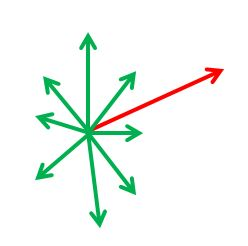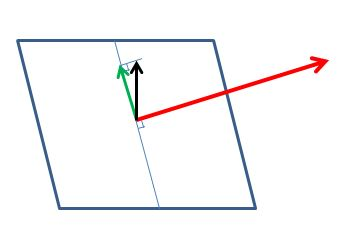# How To Use Path3D

A `Path3D` is a mathematical object created from a sequence of position vectors of points on a curve. Once obtained a Path3D can be used to determine the triplet of vectors tangent, normal and binormal of the curve for each of those points. Each triplet can then be used as a local system coordinate. You could set, for example, the camera direction to the normal as it follows the curve.

A `Path3D` object is simple to construct as follows

``````var points = [v1, v2, ..., vn];          // array of Vector3
var path3d = new BABYLON.Path3D(points);
``````

You can then get the array of tangents, normals and binormals as follows

``````var tangents = path3D.getTangents();
var normals = path3D.getNormals();
var binormals = path3D.getBinormals();
``````

each element of the arrays is a `Vector3` .

Please zoom in and rotate : tangents in red, normals in blue, binormal in green.

Notice, in the next example, how the the triplets slightly rotate when the curve goes more into depth.

Whilst at any point on the curve there is only one tangent there can be an infinite number of normals and hence binormals. If the default one does not suit you it is possible to set the normal direction

## Path3D Methods

As well as `getTangents`, `getNormals` and `getBinormals` there are some other methods of `Path3D`.

### Get Curve Points

The `getCurve` method returns a copy of the initial `Vector3` array given to create the `Path3D` object.

``````var curvePoints = path3d.getCurve();
``````

### Get Distances

The `getDistances` method returns an array of distances from one curve point to the next in order of points and with 0 being the first distance.

For the array of points [(1, 0, 0), (5, 0, 0), (5, 1, 0)] the array of distances is [0, 4, 5].

``````var distances = path3d.getDistances();
``````

### Interpolation

You can get info about any virtual point (from 0.0 to 1.0) along the path by functions that interpolate between the path points. Those are the following:

`getPointAt`, `getTangentAt`, `getNormalAt`, `getBinormalAt`, `getDistanceAt`, `getPreviousPointIndexAt`, `getSubPositionAt`

### Copying (part of) the path

The `slice` method returns a new Path3D that is subpath (slice) of the original path. It takes a start and end position from 0.0 to 1.0, or negative values, which wrap back around from 1.0

### Update

In order to avoid memory re-allocation (when in the render loop for example) since the given points array is internally copied, you can update an existing `Path3D` object with its `update()` method.

``````var points1 = [v1, v2, ..., vn];          // array of Vector3
var path3d = new BABYLON.Path3D(points1);
var points2 = [u1, u2, ..., un];          // another array of Vector3
path3D.update(points2);
``````

Tangents, normals and bi-normals are thus recomputed for this new path.

### Getting the closest position to a point

The `getClosestPositionTo` function returns the position, from 0.0 to 1.0, of the closest virtual point along the path to an arbitrary Vector3.

## Set The Normal

For any vector there are an infinite number of vectors at right angles to it.In creating a `Path3D` object Babylon.js assigns a default direction for the normal. If you want to set the direction of the normal yourself, you need to pass a vector for a second parameter to `Path3D` on creation or on update.

``````var initialVector = new BABYLON.Vector3(0, 1, 0);
var otherVector = new BABYLON.Vector3(0, 0, 1);
var points = [v1, v2, ..., vn];          // array of Vector3
var path3d = new BABYLON.Path3D(points, initialVector);
// do stuff ...
path3d.update(points, otherVector);
``````

The normal will be the projection of your parameter vector onto the plane orthogonal to the tangent at the point position.

As can been in the diagram below, when the parameter is a vertical vector (black), this is projected onto the plane orthoganal to the tangent vector (red) to form the normal (green).The playground example shows what happens as the vector setting the normal direction is rotated.

## Normalization and tangent alignment

Apart from the first normal, there are two more parameters:

`raw`, boolean, false by default. If true the tangents, normals and binormals aren't normalized. Useful to depict path acceleration or speed.

`alignTangentsWithPath`, boolean, false by default. If true the tangents will be aligned with the path.